Courses

# Combinational & Sequential Circuits Computer Science Engineering (CSE) Notes | EduRev

## Computer Science Engineering (CSE) : Combinational & Sequential Circuits Computer Science Engineering (CSE) Notes | EduRev

The document Combinational & Sequential Circuits Computer Science Engineering (CSE) Notes | EduRev is a part of the Computer Science Engineering (CSE) Course GATE Computer Science Engineering(CSE) 2022 Mock Test Series.
All you need of Computer Science Engineering (CSE) at this link: Computer Science Engineering (CSE)

## Combinational Circuits

Combinational circuit is a circuit in which we combine the different gates in the circuit, for example encoder, decoder, multiplexer and demultiplexer. Some of the characteristics of combinational circuits are following −

• The output of combinational circuit at any instant of time, depends only on the levels present at input terminals.

• The combinational circuit do not use any memory. The previous state of input does not have any effect on the present state of the circuit.

• A combinational circuit can have an n number of inputs and m number of outputs.

### Block diagram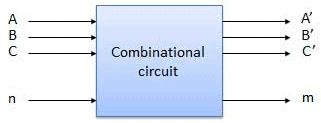We're going to elaborate few important combinational circuits as follows.

## Half Adder

Half adder is a combinational logic circuit with two inputs and two outputs. The half adder circuit is designed to add two single bit binary number A and B. It is the basic building block for addition of two single bit numbers. This circuit has two outputs carry and sum.

### Block diagram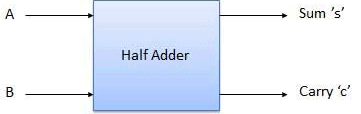### Circuit Diagram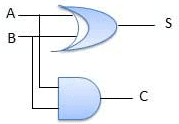## Full Adder

Full adder is developed to overcome the drawback of Half Adder circuit. It can add two one-bit numbers A and B, and carry c. The full adder is a three input and two output combinational circuit.

### Block diagram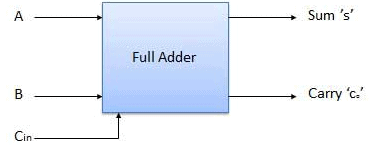### Truth Table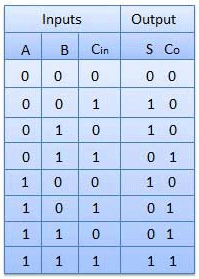### Circuit Diagram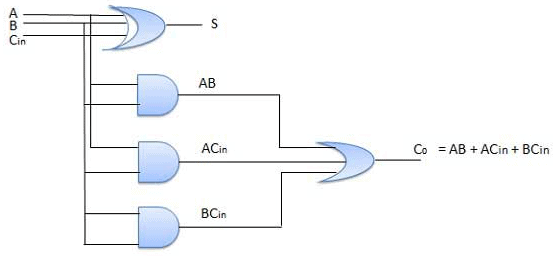## N-Bit Parallel Adder

The Full Adder is capable of adding only two single digit binary number along with a carry input. But in practical we need to add binary numbers which are much longer than just one bit. To add two n-bit binary numbers we need to use the n-bit parallel adder. It uses a number of full adders in cascade. The carry output of the previous full adder is connected to carry input of the next full adder.

### 4 Bit Parallel Adder

In the block diagram, A0 and B0 represent the LSB of the four bit words A and B. Hence Full Adder-0 is the lowest stage. Hence its Cin has been permanently made 0. The rest of the connections are exactly same as those of n-bit parallel adder is shown in fig. The four bit parallel adder is a very common logic circuit.

### Block diagram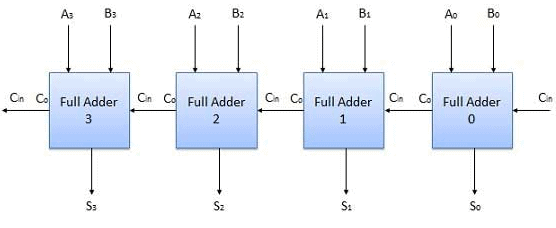## N-Bit Parallel Subtractor

The subtraction can be carried out by taking the 1's or 2's complement of the number to be subtracted. For example we can perform the subtraction (A-B) by adding either 1's or 2's complement of B to A. That means we can use a binary adder to perform the binary subtraction.

### 4 Bit Parallel Subtractor

The number to be subtracted (B) is first passed through inverters to obtain its 1's complement. The 4-bit adder then adds A and 2's complement of B to produce the subtraction. S3 S2 S1 S0 represents the result of binary subtraction (A-B) and carry output Cout represents the polarity of the result. If A > B then Cout = 0 and the result of binary form (A-B) then Cout = 1 and the result is in the 2's complement form.

### Block diagram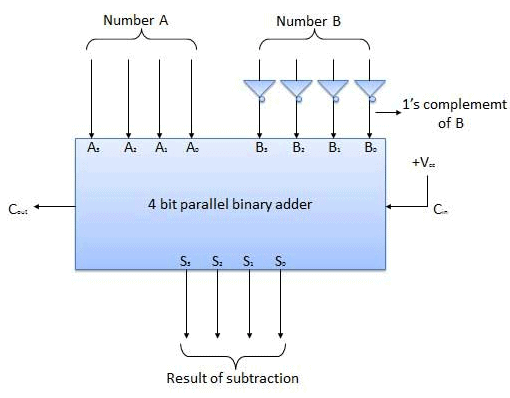## Half Subtractors

Half subtractor is a combination circuit with two inputs and two outputs (difference and borrow). It produces the difference between the two binary bits at the input and also produces an output (Borrow) to indicate if a 1 has been borrowed. In the subtraction (A-B), A is called as Minuend bit and B is called as Subtrahend bit.

### Truth Table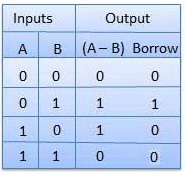### Circuit Diagram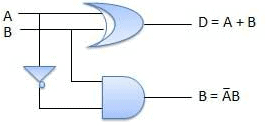## Full Subtractors

The disadvantage of a half subtractor is overcome by full subtractor. The full subtractor is a combinational circuit with three inputs A,B,C and two output D and C'. A is the 'minuend', B is 'subtrahend', C is the 'borrow' produced by the previous stage, D is the difference output and C' is the borrow output.

### Truth Table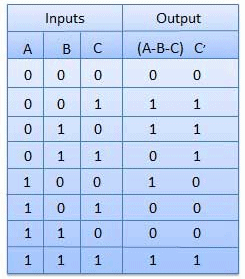### Circuit Diagram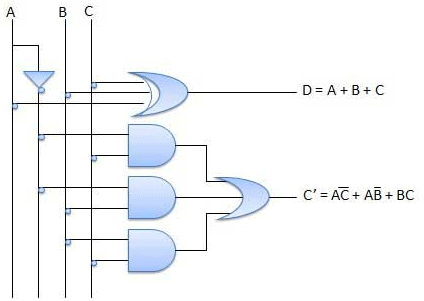## Multiplexers

Multiplexer is a special type of combinational circuit. There are n-data inputs, one output and m select inputs with 2m = n. It is a digital circuit which selects one of the n data inputs and routes it to the output. The selection of one of the n inputs is done by the selected inputs. Depending on the digital code applied at the selected inputs, one out of n data sources is selected and transmitted to the single output Y. E is called the strobe or enable input which is useful for the cascading. It is generally an active low terminal that means it will perform the required operation when it is low.

### Block diagram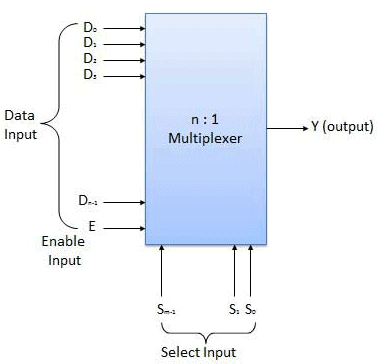Multiplexers come in multiple variations

• 2 : 1 multiplexer
• 4 : 1 multiplexer
• 16 : 1 multiplexer
• 32 : 1 multiplexer

### Block Diagram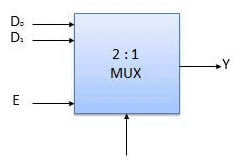### Truth Table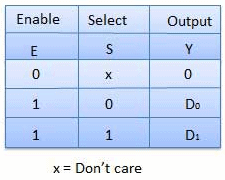## Demultiplexers

A demultiplexer performs the reverse operation of a multiplexer i.e. it receives one input and distributes it over several outputs. It has only one input, n outputs, m select input. At a time only one output line is selected by the select lines and the input is transmitted to the selected output line. A de-multiplexer is equivalent to a single pole multiple way switch as shown in fig.

Demultiplexers comes in multiple variations.

• 1 : 2 demultiplexer
• 1 : 4 demultiplexer
• 1 : 16 demultiplexer
• 1 : 32 demultiplexer

### Block diagram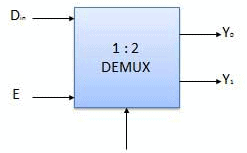### Truth Table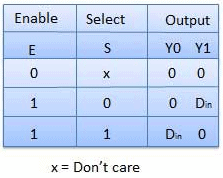## Decoder

A decoder is a combinational circuit. It has n input and to a maximum m = 2n outputs. Decoder is identical to a demultiplexer without any data input. It performs operations which are exactly opposite to those of an encoder.

### Block diagram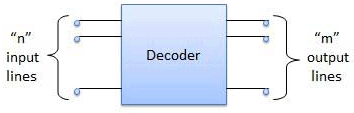Examples of Decoders are following.

• Code converters
• BCD to seven segment decoders
• Nixie tube decoders
• Relay actuator

## 2 to 4 Line Decoder

The block diagram of 2 to 4 line decoder is shown in the fig. A and B are the two inputs where D through D are the four outputs. Truth table explains the operations of a decoder. It shows that each output is 1 for only a specific combination of inputs.

### Block diagram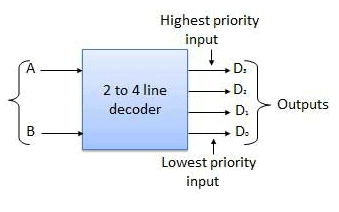### Truth Table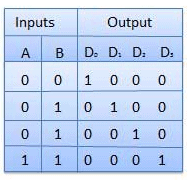### Logic Circuit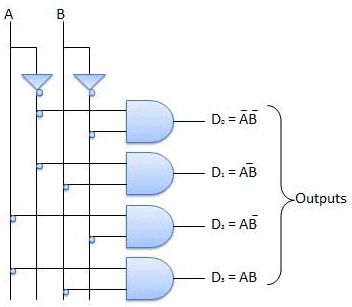## Encoder

Encoder is a combinational circuit which is designed to perform the inverse operation of the decoder. An encoder has n number of input lines and m number of output lines. An encoder produces an m bit binary code corresponding to the digital input number. The encoder accepts an n input digital word and converts it into an m bit another digital word.

### Block diagram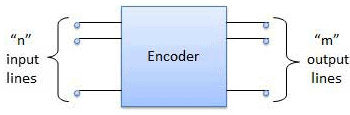Examples of Encoders are following.

• Priority encoders
• Decimal to BCD encoder
• Octal to binary encoder
• Hexadecimal to binary encoder

## Priority Encoder

This is a special type of encoder. Priority is given to the input lines. If two or more input line are 1 at the same time, then the input line with highest priority will be considered. There are four input D0, D1, D2, D3 and two output Y0, Y1. Out of the four input D3 has the highest priority and D0 has the lowest priority. That means if D3 = 1 then Y1 Y1 = 11 irrespective of the other inputs. Similarly if D3 = 0 and D2 = 1 then Y1 Y0 = 10 irrespective of the other inputs.

### Block diagram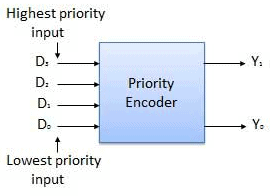### Truth Table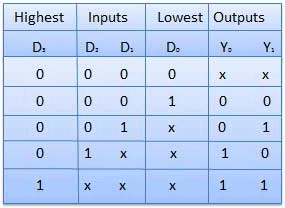### Logic Circuit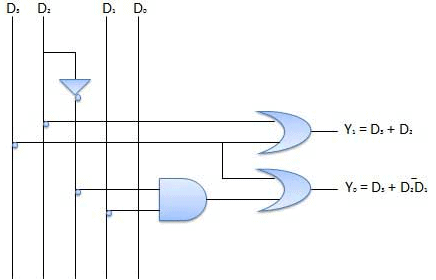## Sequential Circuits

The combinational circuit does not use any memory. Hence the previous state of input does not have any effect on the present state of the circuit. But sequential circuit has memory so output can vary based on input. This type of circuits uses previous input, output, clock and a memory element.

## Block diagram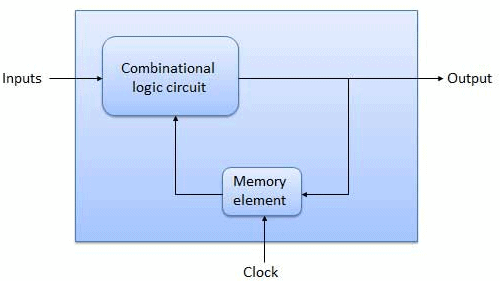## Flip Flop

Flip flop is a sequential circuit which generally samples its inputs and changes its outputs only at particular instants of time and not continuously. Flip flop is said to be edge sensitive or edge triggered rather than being level triggered like latches.

## S-R Flip Flop

It is basically S-R latch using NAND gates with an additional enable input. It is also called as level triggered SR-FF. For this, circuit in output will take place if and only if the enable input (E) is made active. In short this circuit will operate as an S-R latch if E = 1 but there is no change in the output if E = 0.

### Block Diagram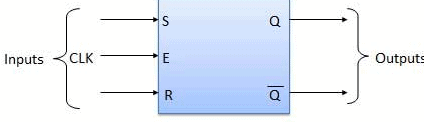### Circuit Diagram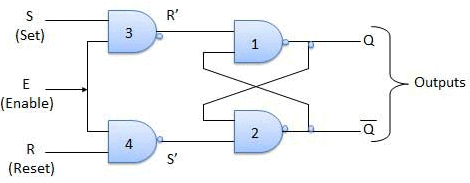### Truth Table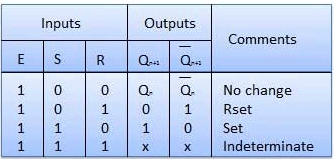### Operation

 S.N. Condition Operation 1 S = R = 0 : No change If S = R = 0 then output of NAND gates 3 and 4 are forced to become 1.Hence R' and S' both will be equal to 1. Since S' and R' are the input of the basic S-R latch using NAND gates, there will be no change in the state of outputs. 2 S = 0, R = 1, E = 1 Since S = 0, output of NAND-3 i.e. R' = 1 and E = 1 the output of NAND-4 i.e. S' = 0.Hence Qn+1 = 0 and Qn+1 bar = 1. This is reset condition. 3 S = 1, R = 0, E = 1 Output of NAND-3 i.e. R' = 0 and output of NAND-4 i.e. S' = 1.Hence output of S-R NAND latch is Qn+1 = 1 and Qn+1 bar = 0. This is the reset condition. 4 S = 1, R = 1, E = 1 As S = 1, R = 1 and E = 1, the output of NAND gates 3 and 4 both are 0 i.e. S' = R' = 0.Hence the Race condition will occur in the basic NAND latch.

## Master Slave JK Flip Flop

Master slave JK FF is a cascade of two S-R FF with feedback from the output of second to input of first. Master is a positive level triggered. But due to the presence of the inverter in the clock line, the slave will respond to the negative level. Hence when the clock = 1 (positive level) the master is active and the slave is inactive. Whereas when clock = 0 (low level) the slave is active and master is inactive.

### Circuit Diagram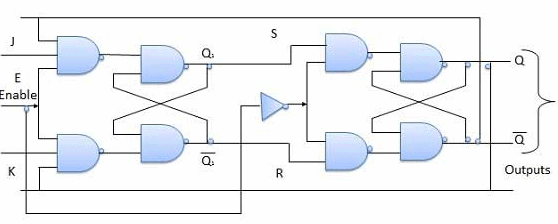Truth Table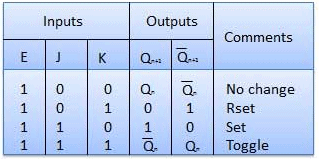### Operation

 S.N. Condition Operation 1 J = K = 0 (No change) When clock = 0, the slave becomes active and master is inactive. But since the S and R inputs have not changed, the slave outputs will also remain unchanged. Therefore outputs will not change if J = K =0. 2 J = 0 and K = 1 (Reset) Clock = 1 − Master active, slave inactive. Therefore outputs of the master become Q1 = 0 and Q1 bar = 1. That means S = 0 and R =1.Clock = 0 − Slave active, master inactive. Therefore outputs of the slave become Q = 0 and Q bar = 1.Again clock = 1 − Master active, slave inactive. Therefore even with the changed outputs Q = 0 and Q bar = 1 fed back to master, its output will be Q1 = 0 and Q1 bar = 1. That means S = 0 and R = 1.Hence with clock = 0 and slave becoming active the outputs of slave will remain Q = 0 and Q bar = 1. Thus we get a stable output from the Master slave. 3 J = 1 and K = 0 (Set) Clock = 1 − Master active, slave inactive. Therefore outputs of the master become Q1 = 1 and Q1 bar = 0. That means S = 1 and R =0.Clock = 0 − Slave active, master inactive. Therefore outputs of the slave become Q = 1 and Q bar = 0.Again clock = 1 − then it can be shown that the outputs of the slave are stabilized to Q = 1 and Q bar = 0. 4 J = K = 1 (Toggle) Clock = 1 − Master active, slave inactive. Outputs of master will toggle. So S and R also will be inverted.Clock = 0 − Slave active, master inactive. Outputs of slave will toggle.These changed output are returned back to the master inputs. But since clock = 0, the master is still inactive. So it does not respond to these changed outputs. This avoids the multiple toggling which leads to the race around condition. The master slave flip flop will avoid the race around condition.

Delay Flip Flop / D Flip Flop

Delay Flip Flop or D Flip Flop is the simple gated S-R latch with a NAND inverter connected between S and R inputs. It has only one input. The input data is appearing at the output after some time. Due to this data delay between i/p and o/p, it is called delay flip flop. S and R will be the complements of each other due to NAND inverter. Hence S = R = 0 or S = R = 1, these input condition will never appear. This problem is avoid by SR = 00 and SR = 1 conditions.

### Block Diagram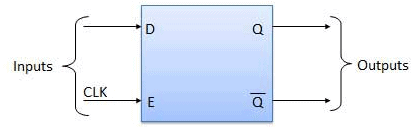### Circuit Diagram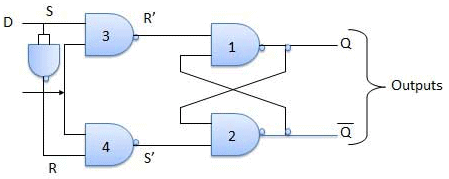### Truth Table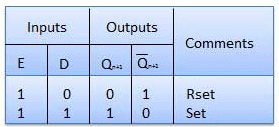### Operation

 S.N. Condition Operation 1 E = 0 Latch is disabled. Hence no change in output. 2 E = 1 and D = 0 If E = 1 and D = 0 then S = 0 and R = 1. Hence irrespective of the present state, the next state is Qn+1 = 0 and Qn+1 bar = 1. This is the reset condition. 3 E = 1 and D = 1 If E = 1 and D = 1, then S = 1 and R = 0. This will set the latch and Qn+1 = 1 and Qn+1 bar = 0 irrespective of the present state.

Toggle Flip Flop / T Flip Flop

Toggle flip flop is basically a JK flip flop with J and K terminals permanently connected together. It has only input denoted by T as shown in the Symbol Diagram. The symbol for positive edge triggered T flip flop is shown in the Block Diagram.

### Symbol Diagram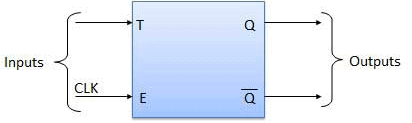### Block Diagram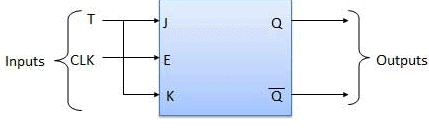### Truth Table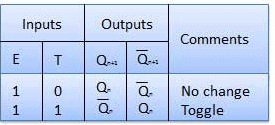### Operation

 S.N. Condition Operation 1 T = 0, J = K = 0 The output Q and Q bar won't change 2 T = 1, J = K = 1 Output will toggle corresponding to every leading edge of clock signal.

Counters in Digital Logic

According to Wikipedia, in digital logic and computing, a Counter is a device which stores (and sometimes displays) the number of times a particular event or process has occurred, often in relationship to a clock signal. Counters are used in digital electronics for counting purpose, they can count specific event happening in the circuit. For example, in UP counter a counter increases count for every rising edge of clock. Not only counting, a counter can follow the certain sequence based on our design like any random sequence 0,1,3,2… .They can also  be designed with the help of flip flops.

Counter Classification

Counters are broadly divided into two categories

1. Asynchronous counter
2. Synchronous counter

1. Asynchronous Counter

In asynchronous counter we don’t use universal clock, only first flip flop is driven by main clock and the clock input of rest of the following counters is driven by output of previous flip flops. We can understand it by following diagram-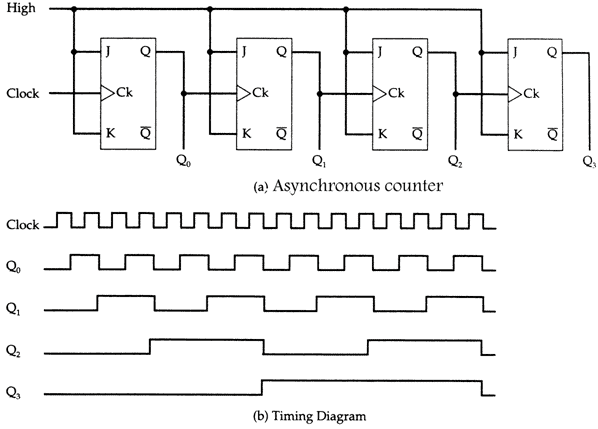It is evident from timing diagram that Q0 is changing as soon as the rising edge of clock pulse is encountered, Q1 is changing when rising edge of Q0 is encountered(because Q0 is like clock pulse for second flip flop) and so on. In this way ripples are generated through Q0,Q1,Q2,Q3 hence it is also called RIPPLE counter.

2. Synchronous Counter

Unlike the asynchronous counter, synchronous counter has one global clock which drives each flip flop so output changes in parallel. The one advantage of synchronous counter over asynchronous counter is, it can operate on higher frequency than asynchronous counter as it does not have cumulative delay because of same clock is given to each flip flop.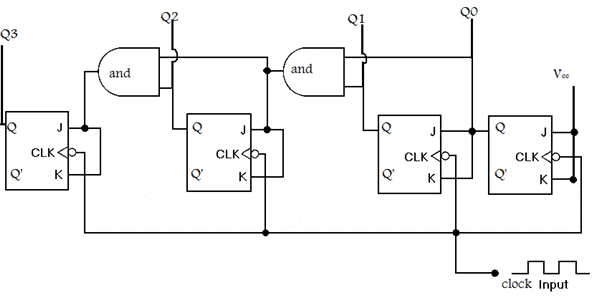Synchronous  counter circuit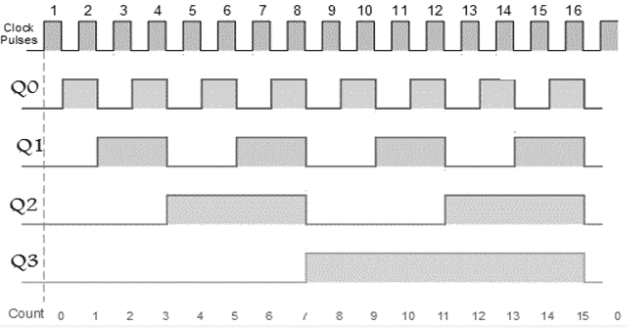Timing diagram synchronous counter

From circuit diagram we see that Q0 bit gives response to each falling edge of clock while Q1 is dependent on Q0, Q2 is dependent on Q1 and Q0 , Q3 is dependent on Q2,Q1 and Q0.

Decade Counter

A decade counter counts ten different states and then reset to its initial states. A simple decade counter will count from 0 to 9 but we can also make the decade counters which can go through any ten states between 0 to 15(for 4 bit counter).

 Clock pulse Q3 Q2 Q1 Q0 0 0 0 0 0 1 0 0 0 1 2 0 0 1 0 3 0 0 1 1 4 0 1 0 0 5 0 1 0 1 6 0 1 1 0 7 0 1 1 1 8 1 0 0 0 9 1 0 0 1 10 0 0 0 0

Truth table for simple decade counter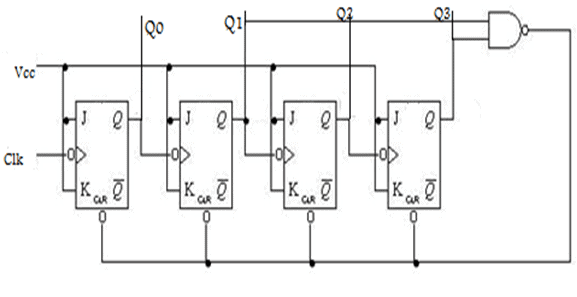Decade counter circuit diagram

We see from circuit diagram that we have used nand gate for Q3 and Q1 and feeding this to clear input line because binary representation of 10 is—

1010

And we see Q3 and Q1 are 1 here, if we give NAND of these two bits to clear input then counter will be clear at 10 and again start from beginning.

Important point: Number of flip flops used in counter are always greater than equal to (log2 n)  where n=number of states in counter.

Some previous years gate questions on Counters

Consider the partial implementation of a 2-bitt counter using T flip-flops following the sequence 0-2-3-1-0, as shown below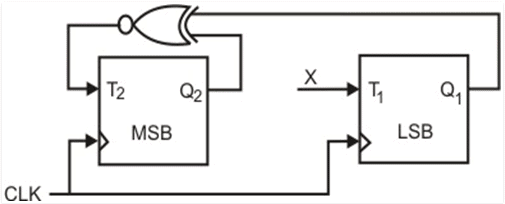To complete the circuit, the input X should be

(A) Q2′
(B) Q2 + Q1
(C) (Q1 ⊕ Q2)’
(D) Q1 ⊕ Q2

Solution:

From circuit we see

T1=XQ1’+X’Q1—-(1)

AND

T2=(Q2 ⊕ Q1)’—-(2)

AND DESIRED OUTPUT IS 00->10->11->01->00

SO X SHOULD BE Q1Q2’+Q1’Q2 SATISFYING 1 AND 2.

SO ANS IS (D) PART.

The control signal functions of a 4-bit binary counter are given below (where X is “don’t care”)
The counter is connected as follows: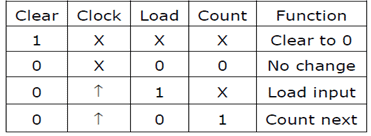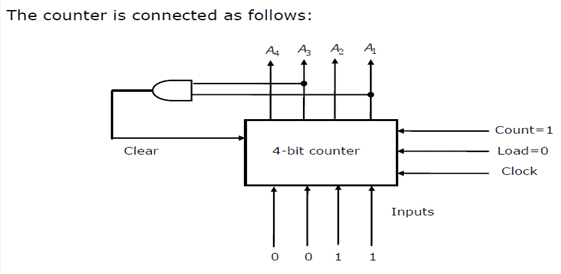Assume that the counter and gate delays are negligible. If the counter starts at 0, then it cycles through the following sequence:

(A) 0,3,4

(B) 0,3,4,5

(C) 0,1,2,3,4

(D) 0,1,2,3,4,5

Solution:

Initially A1 A2 A3 A4 =0000

Clr=A1 and A3

So when A1 and A3 both are 1 it again goes to 0000

Hence 0000(init.) -> 0001(A1 and A3=0)->0010 (A1 and A3=0) -> 0011(A1 and A3=0) -> 0100 (A1 and A3=1)[ clear condition satisfied] ->0000(init.) so it goes through 0->1->2->3->4

Ans is (C) part.

Offer running on EduRev: Apply code STAYHOME200 to get INR 200 off on our premium plan EduRev Infinity!

## GATE Computer Science Engineering(CSE) 2022 Mock Test Series

131 docs|167 tests

,

,

,

,

,

,

,

,

,

,

,

,

,

,

,

,

,

,

,

,

,

;